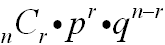Bernoulli Trials -
Binomial Probability Distribution

The formula used with Bernoulli trials computes the binomial probability of obtaining
exactly "r" events in "n" trials:n = number of trials   r = number of specific events you wish to obtain   p = probability that the event will occur   q = probability that the event will not occur         (q = 1 - p, the complement of the event)

If you enter the formula directly on the home screen, be careful to use parentheses when entering the exponent of  n - r  (or do the subtraction mentally and enter your calculation).

 Consider a problem where n = 6, r = 3, and p = 50% (so, p = .5 and q = .5, where  q = 1 - p) (Remember, the function nCr is found under MATH → PRB #3 nCr and requires that the first value, n, be entered before the function is called.)The easiest way to utilize the calculator to solve this formula is to engage the binompdf function:

 binompdf( binomial distribution probability density function, which is:where(When using this built-in function there is no need to     type in the formula - YEA!!)
 Consider, again, a problem where n = 6, r = 3, and p = 50% (Remember, the function binompdf is found under DISTR (2nd VARS), arrow down to #0 binompdf and the parameters are:  binompdf (number of trials, probability of occurrence, number of specific events)Finding Your Way Around TABLE of  CONTENTS